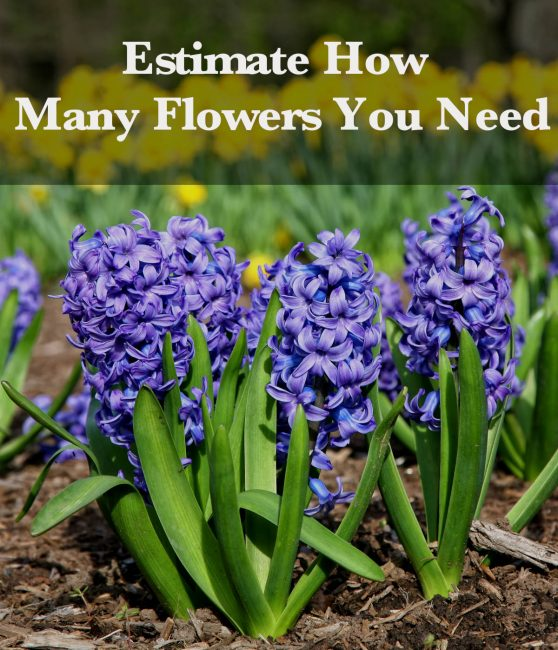# Plant Spacing Calculator – Find How Many Plants You Need

Estimate the number of plants or flowers needed to fill a landscape bed by entering the size of the bed and the desired plant spacing. The calculator will show the number of plants needed using various layout patterns.

## Plant Estimate:

Rectangular Pattern

plantsTriangular Pattern

plantsPlanting Area

Ft2
Learn how we calculated this below

## How to Calculate How Many Plants You Need

The first step to finding how many plants or flowers you need is to select a pattern you would like to use when planting them and choose the spacing between each plant. Plants may be put in a rectangular or a triangular grid and estimating the plants needed for each pattern is a bit different.

### Rectangular Planting Grid

To plant flowers in a rectangular pattern, place each in parallel rows and columns in a grid. Start by dividing the landscape bed into squares that are the width and length of the desired spacing, then add a plant at each corner of the square.

To estimate how many plants are needed, find the number of rows and columns and determine the spacing between each.#### Steps to Estimate Plant Spacing for a Rectangular Grid

First, measure the landscape bed length and width in inches. Use our feet to inches conversion tool to convert a measurement in feet to inches.

Divide the width by the spacing to get the number of columns then divide the length by the spacing to get the number of rows.

Finally, multiply the rows by the columns to find the number of plants needed.

### Triangle Planting Grid

Planting flowers in a triangle pattern is a little bit different. It involves offsetting each row so that the plants are at a 45° angle to each other.

To plant using the triangle pattern, divide the landscape bed into a series of equilateral triangles with the edges of the triangle the desired spacing. The rows will be offset or shifted by about half of the spacing length and will be closer together since the spacing will be at an angle.#### Steps to Estimate Plant Spacing for a Triangle Grid

To estimate the number of plants needed, measure the length and width of the landscape bed in inches.

Then, divide the width by the plant spacing to find the number of columns.

Since the plants are at an angle to each other, the spacing between the rows will be shorter. Use the following formula to find the spacing between rows:

row spacing = (plant spacing ÷ 2) × √3

To find the number of rows simply divide the length by the row spacing.

Finally, multiply the number of rows by the number of columns to find how many plants are needed.

Check out our soil calculator to estimate soil for your plantings and our mulch calculator to find how much mulch you need for your landscaping.